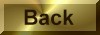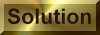Practice Problem 1

Determine the oxidation number of each element in the following compounds:

(a) BaO2     (b) (NH4)2MoO4      (c)Na3Co(NO2)6      (d) CS2

(a)  Ba is +2 and O is -1.

(b) H is +1, N is -3, Mo is -6, and O is -2.

(c) N is +3, O is -2, and Co is +3.

(d) S is -2 and C is +4.